# Statistical Arbitrage

## Description

Statistical Arbitrage (SA) is build to gain profit on simultaneously buying and selling two shares of two correlated instruments. It is recomended to find two securities that are in the same sector / industry, they should have similar market capitalization and average volume traded. SA Strategy calculates two following values, which are later used to generate proper trade signals:

• Mean (M) ratio of the first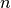periods prices of two assets
• Standard deviation (SD) of ratio of the firstperiods prices of two assets

whereis a Number of Periods.

Mean and standard deviation are defined as follows: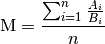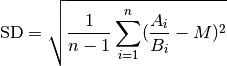where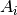(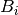) is a price of the first (second) asset at the moment.

SA strategy starts decision rule, if orders should be sent after receiving Number of Periods trades.

• Latest Tick

## Parameters

PARAMETER NAME DESCRIPTION ESSENTIAL
Number of Periods Number of last ‘last trades’ events taken into account in a computation of the mean and standard deviation Yes

## Conditions

### Open position

Amount One asset per order
Price Last market price
Type Market

Consider an arbitrary moment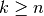, when strategy has no opened positions. If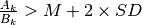then we sell asset A and buy B. Similarly when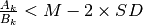then we buy asset A and sell B.

### Close position

Consider an arbitrary moment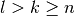, when strategy has already some opened positions. If we buy one asset and sell the other at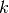we should close these two positions when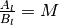.

### Termination

There is no strategy termination condition.

### Time frame

This strategy is dedicated to run in a long period of time. The instruments are highly correlated, so it is not likely that the ratio of prices will differ from mean by two standard deviations.

## Further information & source

• Barry Johnson, Algorithmic Trading & DMA: An introduction to direct access trading strategies, 4Myeloma Press, 2010.